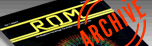`ROM MAGAZINE ISSUE 4 — FEBRUARY/MARCH 1984 / PAGE 20`

 YELLOW BRICK ROAD By PETER ELLISON     In this installment of "The Yellow Brick Road" I'm going to have a brief look at ATARI'S sound. Sound on the Atari is so easy to use because even a BASIC programmer can access it very easily. A sound can be produced so easily by typing in SOUND #1,#2,#3,#4 where #1 means the voice(Atari has four voices 0-3), and #2 is the pictch(from 1-255), #3 the distortion(0-14 even numbers only), and #4 the volume(1-15 and 0 for rest or off).     By varying what numbers are put in these four spaces some very imaginative sounds can be made. From making it sound like a piano to having it sound like a machine gun these can all be done in Basic. The first demo sound is one of a bird. By varying the pitch(X) from 1 to 14 makes it sound like birds are singing. By changing the distortion these birds can be made to sound like guns. 5 REM BIRDS SINGING 10 A=1:C=14:D=10 15 FOR B=1 TO 14 20 SOUND A,B,C,D:NEXT B 30 GOTO 15 By changing C in line 10 to a 0 the sound becomes like a train or by changing the distortion and pitch you can make it sound like you have car trouble like in the next program. 5 REM CAR TROUBLE? 10 A=1:C=2:D=10 15 FOR B=1 TO 20 20 SOUND A,B,C,D:NEXT B 30 GOTO 15 Until now I have only used one loop inorder to produce a sound. In the next little program two loops are used to make a sound like a Spaceship Lift-off. 10 REM SPACESHIP LIFT-OFF OR HYPERJUMP 15 A=0:C=8:D=12 20 FOR B=255 TO 1 STEP -4 30 SOUND A,B,C,D 40 GOSUB 70 50 NEXT B 60 END 70 FOR X=1 TO 50:NEXT X:RETURN We have only used voice number 1 so far but in this next program it gives an example of using all four voices inorder to produce a sound. These four voices can also be used to play music in four-part harmony. 5 REM ALL FOUR VOICES 10 SOUND X=1 TO 100 20 SOUND 0,X,10,10 30 SOUND 1,X+2,10,10 40 SOUND 2,100-X,10,10 50 SOUND 3,2*X,10,10 60 FOR W=1 TO 100:NEXT W 70 NEXT X The final program is a four voice sound editor that uses four joysticks(one for each voice). First you must enter the volume for each voice and then with you Joystick push it up or down, left or right to change either the distortion or pitch. Hold down the Joystick button for the voice that you wish to hear or push all buttons at once to get all four voices. 10 REM SOUND EDITOR 20 REM 25 ? "INPUT VOLUME FOR EACH VOICE #1,#2,#3,#4(1-15)";:INPUT C,C1,C2,C3 30 IF STICK(0)=14 THEN A=A+1:IF A>255 THEN A=1 35 IF STICK(0)=13 THEN A=A-1:IF A<0 THEN A=255 40 IF STICK(0)=11 THEN B=B+2:IF B>14 THEN B=0 45 IF STICK(0)=7 THEN B=B-2:IF B<0 THEN B=14 46 IF STRIG(0)=0 THEN SOUND 0,A,B,C 47 IF STRIG(0)=1 THEN SOUND 0,0,0,0 50 IF STICK(1)=14 THEN A1=A1+1:IF A1>255 THEN A1=1 56 IF STICK(1)=13 THEN A1=A1+1:IF A1<0 THEN A1=255 57 IF STICK(1)=11 THEN B1=B1+2:IF B1>14 THEN B1=0 58 IF STICK(1)=7 THEN B1=B1-2:IF B1<0 THEN B1=14 59 IF STRIG(1)=0 THEN SOUND 0,A1,B1,C1 60 IF STRIG(1)=0 THEN SOUND 0,0,0,0 61 IF STICK(2)=14 THEN A2=A2+1:IF A2>255 THEN A2=1 66 IF STICK(2)=13 THEN A2=A2+1:IF A2<0 THEN A2=255 67 IF STICK(2)=11 THEN B2=B2+2:IF B2>14 THEN B2=0 68 IF STICK(2)=7 THEN B2=B2-2:IF B2<0 THEN B2=14 69 IF STRIG(2)=0 THEN SOUND 0,A2,B2,C1 70 IF STRIG(2)=1 THEN SOUND 0,0,0,0 71 IF STICK(3)=14 THEN A3=A3+1:IF A3>255 THEN A3=1 76 IF STICK(3)=13 THEN A3=A3+1:IF A3<0 THEN A3=255 77 IF STICK(3)=11 THEN B3=B3+2:IF B3>14 THEN B3=0 78 IF STICK(3)=7 THEN B3=B3-2:IF B3<0 THEN B3=14 79 IF STRIG(3)=0 THEN SOUND 0,A3,B3,C1 80 IF STRIG(3)=1 THEN SOUND 0,0,0,0 90 POSITION 1,1:? "V0=PIT:";A;"DIS:";B:POSITION 1,3:? "V1=PIT:";A1;"DIS:";B1 95 POSITION 1,5:? "V2=PIT";A2;"DIS:";B2:POSITION 1,7:? "V3=PIT:";A3;"DIS:";B3 200 GOTO 30 Remember if you have any suggestions on what type of locations you would like looked at drop us a line.